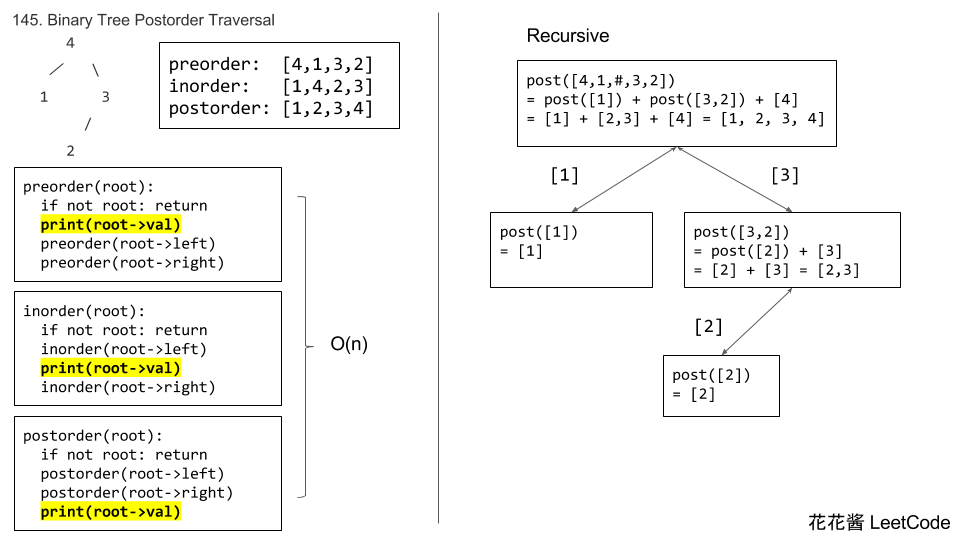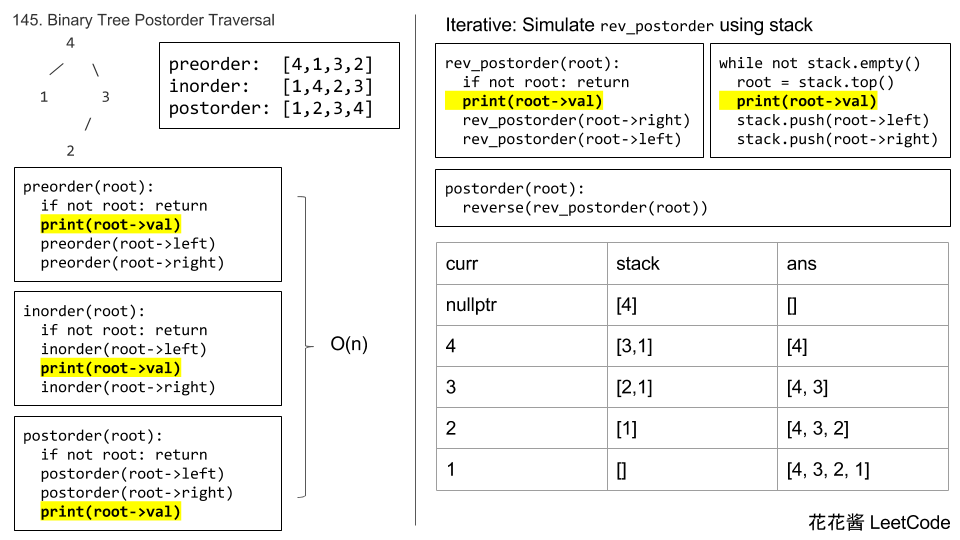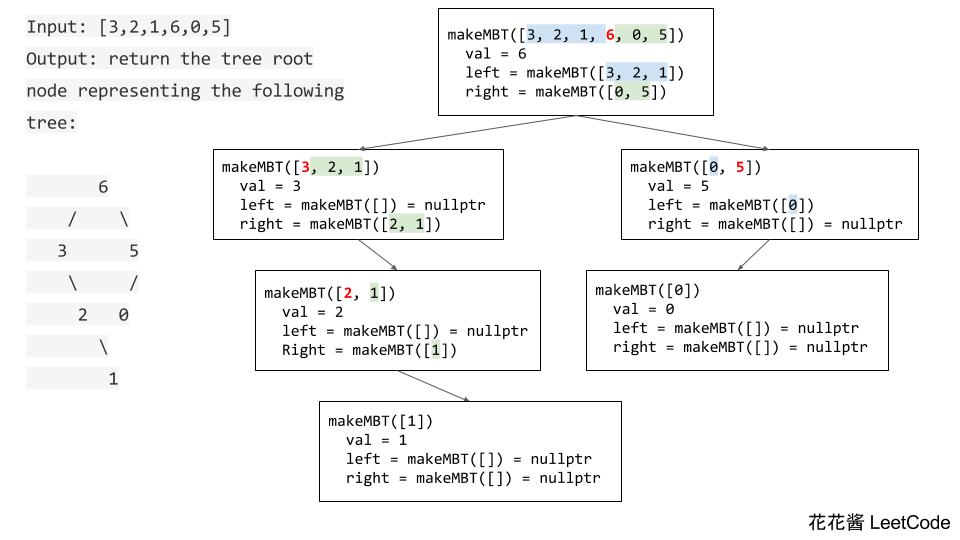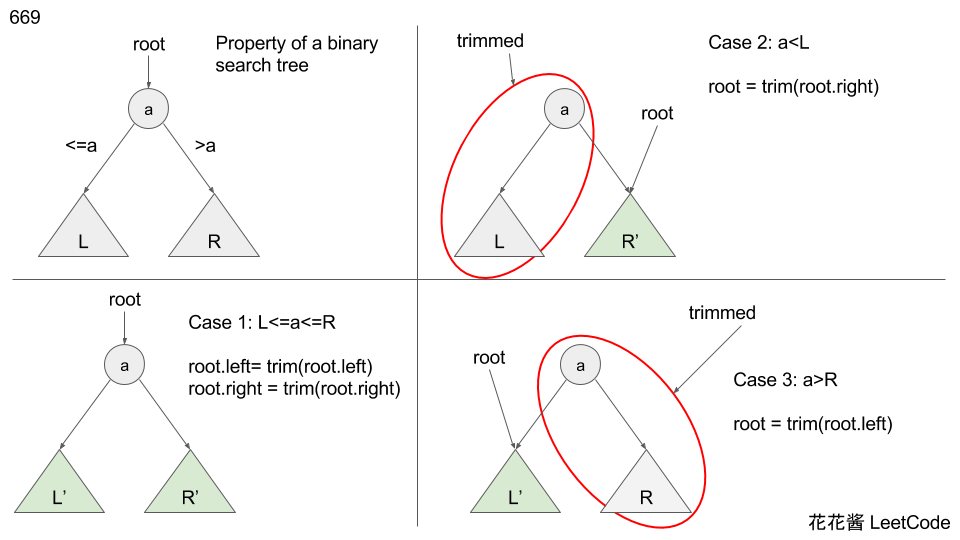# Posts tagged as “binary tree”

Problem:

https://leetcode.com/problems/house-robber-iii/description/

The thief has found himself a new place for his thievery again. There is only one entrance to this area, called the “root.” Besides the root, each house has one and only one parent house. After a tour, the smart thief realized that “all houses in this place forms a binary tree”. It will automatically contact the police if two directly-linked houses were broken into on the same night.

Determine the maximum amount of money the thief can rob tonight without alerting the police.

Example 1:

     3
/ \
2   3
\   \
3   1


Maximum amount of money the thief can rob = 3 + 3 + 1 = 7.

Example 2:

     3
/ \
4   5
/ \   \
1   3   1


Maximum amount of money the thief can rob = 4 + 5 = 9.

Idea:

Compare grandparent + max of grandchildren(l.l + l.r + r.l + r.r) vs max of children (l + r)

Solution 1: Recursion w/o memorization

Time complexity: O(n^2)

Space complexity: O(n)

C++

Solution 2: Recursion w/ memorization

Time complexity: O(n)

Space complexity: O(n)

Solution 3: Recursion return children’s value

Python3

Related Problems:

# Problem:

Given a binary tree, return the postorder traversal of its nodes’ values.

For example:
Given binary tree {1,#,2,3},

return [3,2,1].

Note: Recursive solution is trivial, could you do it iteratively?# Solution 3:

Problem:

Given a non-empty binary tree, return the average value of the nodes on each level in the form of an array.

Example 1:

Time Complexity:

O(n)

Space Complexity:

O(h)

Solution 1:

BFS

Solution 2:

DFS

Related Problems:

Given an integer array with no duplicates. A maximum tree building on this array is defined as follow:

1. The root is the maximum number in the array.
2. The left subtree is the maximum tree constructed from left part subarray divided by the maximum number.
3. The right subtree is the maximum tree constructed from right part subarray divided by the maximum number.

Construct the maximum tree by the given array and output the root node of this tree.

Example 1:

Idea:

RecursionSolution:

With copy

Time complexity: O(nlogn) ~ O(n^2)

Space complexity: O(nlogn) ~ O(n^2)

running time 79ms

Without copy

Time complexity: O(nlogn) ~ O(n^2)

Space complexity: O(logn) ~ O(n)

running time 66ms

Given a binary search tree and the lowest and highest boundaries as L and R, trim the tree so that all its elements lies in [L, R] (R >= L). You might need to change the root of the tree, so the result should return the new root of the trimmed binary search tree.

Example 1:

Example 2:

This problem can be solved with recursionThere 3 cases in total depends on the root value and L, R

Time complexity: O(n)

Space complexity: O(1)

Solution:

The previous solution has potential memory leak for languages without garbage collection.

Here’s the full program to delete trimmed nodes.

Example output

Mission News Theme by Compete Themes.